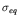Computational & Technology Resources an online resource for computational,engineering & technology publications not logged in - login Civil-Comp ProceedingsISSN 1759-3433 CCP: 83PROCEEDINGS OF THE EIGHTH INTERNATIONAL CONFERENCE ON COMPUTATIONAL STRUCTURES TECHNOLOGY Edited by: B.H.V. Topping, G. Montero and R. Montenegro Paper 166Numerical Modelling of Mechanical Tests Using the Discrete Element Method M. Guessasma1, J. Fortin2 and E. Bellenger11Laboratoire de Technologies Innovantes, IUT de l'Aisne, Saint-Quentin, France 2INSSET de Saint-Quentin, University of Picardie Jules Verne, Saint-Quentin, France doi:10.4203/ccp.83.166 Full Bibliographic Reference for this paper M. Guessasma, J. Fortin, E. Bellenger, "Numerical Modelling of Mechanical Tests Using the Discrete Element Method", in B.H.V. Topping, G. Montero, R. Montenegro, (Editors), "Proceedings of the Eighth International Conference on Computational Structures Technology", Civil-Comp Press, Stirlingshire, UK, Paper 166, 2006. doi:10.4203/ccp.83.166 Keywords: granular medium, mean stress, non-smooth contact dynamics. Summary The aim of this work is to obtain a mean stress tensor of an equivalent continuous medium divided into elementary cells. The cell stress tensor is obtained by considering that it is integrable on a surface. General balance principles imply that such a tensor has convenient properties to build a mean stress tensor (translation invariant, symmetric and additive). A simple analytical example of a cylindrical rigid bead rolling by gravity on an inclined plane is considered. It is shown that taking account of the inertia forces is essential to ensure the symmetry of the bead mean stress. The stress is the product of the density, the gravity acceleration, the granulometry and the plane inclination. The explicit calculation leads to an additive decomposition of stress in a solid part and a fluid part. The simple analytic example illustrates well the necessity to take into account the body forces in dynamics, to obtain a stress tensor in the sense of Cauchy We propose then to calculate the mean stress tensor so defined for a great number of particles for an ensiled material. The calculations are carried out with the software MULTICOR . This software is based on the contact dynamics method. The interaction between particles is described by the unilateral contact law with Coulomb's friction, written with the bipotential concept . We obtain an algorithm of resolution based on a predictor-corrector scheme by projection cone, and a convergence criterion based on the estimator of the relative error in the constitutive law  The simulation which we considered is made in a square box whose upper side is open. We consider that the behaviour of the discrete material is perfectly rigid with density equal to 7800kg/m3 and friction coefficient about 0.3. The granular material contains about 5000 particles with radii of 0.5, 0,7 and 1mm. After sedimentation, by submitting the granular material only to the gravity force, the granular material is then submitted to a punching test of duration 0.3s with a speed of 0.05m/s. By determining the equivalent stressesclassically used in geomechanics, we studied the distribution ofin the granular material and the influence of the penetration depth of the punch. In conclusion, we have proposed in this paper a consistent definition of the mean stress tensor for granular media, which takes into account, not only the contact forces, but also the body forces acting at the grain level. The mean stress tensor so defined has all the properties of a classical stress tensor of a continuum Cauchy material (symmetry and invariance by translation). Moreover the definition proposed is well adapted to numerical calculations. References 1 J. Fortin, Simulation numérique de la dynamique des systèmes multicorps appliquée aux milieux granulaires, Thèse de doctorat de l'Université de Lille-I, 2000. 2 G. De Saxce, Z.Q. Feng, "The bipotential method: a constructive approach to design the complete contact law with friction and improved numerical algorithms", Int. J. Math. Comput. Model. 28 (4-8), 225-245, 1998. purchase the full-text of this paper (price £20) Back to top ©Civil-Comp Limited 2020 - terms & conditions